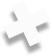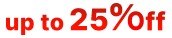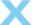We are committed to providing fast, efficient, and affordable software solutions that set new standards in the software development industry.# Data Formats and Multipliers

You may enter data in all numerical fields either in sectors or in bytes. If there is no letters are after the number, R-Linux assumes the numbers are in bytes.

Decimal numbers are entered as they are: 2372354

Hexadecimal numbers are entered as 0x23Fa67 or 23Fa67 hex .

The following case-insensitive notation is possible:

 b 1 byte kb 1 kb = 2^10=1024 bytes mb 1 mb = 2^20=104857 bytes gb 1 gb = 2^30=1073741824 bytes tb 1 tb = 2^40=1099511627776 bytes eb 1 eb = 2^50=1125899906842624 bytes hex A hexadecimal number sec (sector) A number is in sectors

You may also slect the multipliers in the drop-down boxes .

When the multiplier has been changed, the data value will be changed according to the specific context commands for that field:

Suppose, the initial value is 1 GB, and the sector size of the object is 512 byte. The results will be the following:

### Command: No recalculate

Change from GB to MB: 1

Change from GB to Sectors: 1

### Command: Always recalculate

Change from GB to MB: 1024

Change from GB to Sectors: 2097152

### Command: Units type recalculate

Change from GB to MB: 1

Change from GB to Sectors: 2097152